## Proving "for all" statements

### Quick description

This page is an index of general methods for proving statements that begin with a universal quantifier. It needs to be improved and expanded, and the not yet active linked articles need to be written.

Method 1: Convert "every" into a single arbitraryQuick description ( All this means is that if you are trying to prove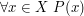then you begin by writing "Let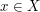," and you then try to prove that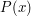, perhaps ending by writing, "Sincewas arbitrary, the proof is complete." For example, if you are asked to prove that every group of prime order is cyclic, then you begin by writing "Let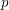be a prime and let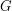be a group of order." You then proceed as thoughwere a fixed number. )

Method 2: Induction Quick description ( If the statement is equivalent to a statement of the form "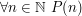" then consider using induction in one of its various forms. For example, the statement "Every positive integer can be written as a product of primes" can be proved by starting with the line "Letbe a positive integer and suppose that every positive integer less thancan be written as a product of primes." )

Method 3: Classification Quick description ( If you find it hard to prove the statementfor an abstract elementof a set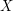, then all is not necessarily lost: you may be able to classify the elements ofand provefor each one. For example, if your statement is of the form "Every finite simple group has property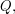" then you may find that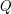does not follow straightforwardly from the simplicity assumption, but that it can be checked for any group that belongs to one of the infinite families of finite simple groups, and also for all the 26 sporadic groups. One could regard such a proof as starting with the line "Letbe a finite simple group," and continuing with "By the classification of finite simple groups,must be one of the following groups." But the first of these two lines is not terribly helpful. This page is an annotated index of Tricki articles about different classification theorems and how they can be used. )

Method 4: Prove the result for some cases and deduce it for the rest Quick description ( It is often possible to establish a result for all objects in a collection by first establishing it for certain cases and then deducing the general result from these cases. This page gives links to various techniques of this general kind. )

### General discussion

Note that induction is a special case of method 1: in both cases we avoid having to write out a separate proof for everyby writing a single proof for a variable. Because of this, every statement that begins with a string of quantifiers can be made to feel as though what it needs is a proof of existence. For example, if we are asked to prove from first principles that the function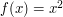is continuous, then we are asked to prove that for everyand every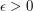there exists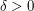such that if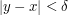then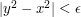. If we start by writing "Letbe a real number and let", then the problem becomes one of proving the existence of a positive numberwith a certain property. Of course, this property depends onand, as does. Another way of thinking of the problem as an existence problem is to say that we are trying to find a functionof two variablesandsuch that whenever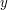andare real numbers with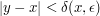we have.

For this reason, the Tricki will concentrate far more on proofs of existence than on proofs of "for all" statements. However, there are some universal problems that do not convert so easily into existence problems, and there are also problems that are not naturally thought of as beginning with either an existential quantifier or a universal one, so the Tricki will not be devoted solely to existence problems.

### Different styles of "for all" problem

This following pages are about universal statements of different common types.

Deducing one property from another Quick description ( Many mathematical theorems take the form "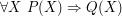", whereis some mathematical structure and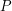andare two properties thatcan have. For instance, "Every group of prime order is cyclic" comes into this category. There is no single method for proving all statements of this kind, but this article is a page with links to articles about solving various classes of them. )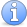Incomplete This article is incomplete. It would be good to have more categories of problem and some kind of conclusion to this article.